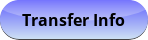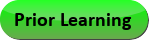Sep 21, 2023
2023-2024 College Catalog
 Select a Catalog 2023-2024 College Catalog 2022-2023 College Catalog [ARCHIVED CATALOG] 2021-2022 College Catalog [ARCHIVED CATALOG] 2020-2021 College Catalog [ARCHIVED CATALOG] 2019-2020 College Catalog [ARCHIVED CATALOG] *** DRAFT 2024-2025 College Catalog DRAFT *** [ARCHIVED CATALOG]
 HELP 2023-2024 College Catalog Print-Friendly Page (opens a new window) Add to Portfolio (opens a new window)

# GTM 105V - Applied Technical Mathematics for Aviation

3 Credits, 3 Contact Hours
3 lecture periods 0 lab periods

Applied geometry and trigonometry operations. Includes review of basic math operations, charts and graphs, review of pre-algebra, elements of geometry, plane trigonometry, and aviation practical applications.

Prerequisite(s): ICS 081  or MAT 086 or completion of Module 15 in MAT 089A  or satisfactory score on the Mathematics assessment test.
Gen-Ed: Meets CTE - M&S.Course Learning Outcomes
1. Demonstrate proficiency performing basic math operations
2. Demonstrate proficiency performing power, root, and ratio calculations
3. Demonstrate proficiency performing area and volume calculations
4. Demonstrate proficiency performing aircraft weight and balance calculations

Outline:
1. Review of Basic Math Operations (CLO 1, 2, 3, 8)
1. Whole/signed numbers
2. Subtract
3. Multiply
4. Divide
2. Fractions
2. Subtract
3. Multiply
4. Divide
3. Decimals
2. Subtract
3. Multiply
4. Divide
4. Metric/English conversions
1. Multiples and prefixes
2. Conversion tables
5. Proportions and ratios
1. Aspect ratio
3. Proportion
6. Formulas
7. Percentages
1. Determining a number which is a percentage of another
2. Determining the percentage that one number is of another
3. Finding the total number when part of the number and its percentage are known
8. Aviation applications of basic math operations / weight and balance
1. Terms and definitions
2. Effective of CG to an aircraft
3. Calculations
4. Corrections
2. Charts and Graphs (CLO 7, 9)
1. Pie charts
2. Broken line graphs
3. Bar graphs
4. Continuous curve graphs
5. Aviation applications of charts and graphs
3. Review of Pre-Algebra (CLO 4, 5)
1. Order of operations
2. Variables
3. Formulas
4. Powers / raising a number to a given power
5. Roots / extracting the root of a number
6. Aviation applications of pre-algebra concepts / bend allowance
1. Flat pattern layout
2. Calculations
4. Elements of Geometry (CLO 6, 9)
1. Angles
1. Measurement
2. Practical application
3. Conversion
4. Complement/supplement
2. Measure of an area
1. Area of a rectangle
2. Areas of a square
3. Area of a triangle
4. Area of a parallelogram
5. Area of a trapezoid
6. Area of a circle
3. Measure of volume
1. Volume of a rectangle
2. Volume of a cube
3. Volume of a cylinder
4. Volume of a sphere
4. Triangles
1. Types
2. Pythagorean theorem
3. Congruent
4. Oblique
5. Aviation applications of geometry
5. Plane Trigonometry (CLO 9)
1. Right triangles
2. Sine, cosine, and tangent
3. Determining angles and sides
4. Area and perimeter

Aviation applications of plane trigonometry

Effective Term: